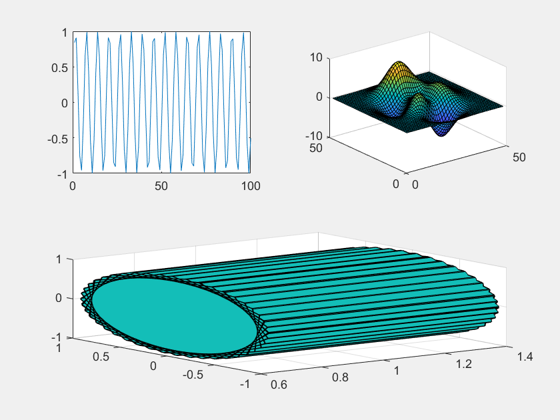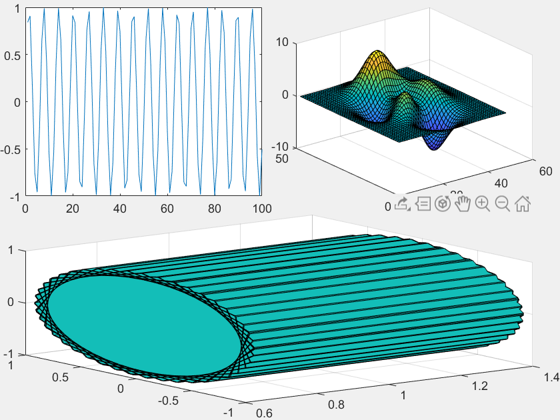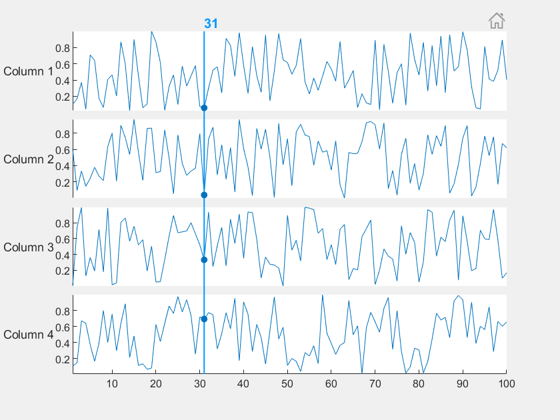# Tiled Layout

Sean‘s pick this week is tiledlayout by MathWorks’s development team.

Check the Release Notes for all of the updates.

### subplot’s Replacement

I imagine most of you have probably used subplot at some point.

Subplot alternatives or extensions are very popular on the File Exchange. In fact, I think it may be the most popular topic of contributions to the FEX. A quick search reveals many of the pains associated with subplots:

• Too much spacing between them
• Too much padding on the edges
• Difficulty in arranging the axes based on figure size
• Scaling issues

Enter R2019b, and tiledlayout. Tiled layouts give us all of the above. Let’s look at two examples: minimizing whitespace, and resizing/rescaling.

### Minimize Whitespace

Create a tiledlayout, and use nexttile to traverse it.

figure
tlt = tiledlayout(2, 2);
nexttile
plot(sin(1:100));

nexttile
surf(peaks)

nexttile([1 2]) % Spanned
ribbon(sin(1:100), cos(1:100))And let’s get rid of that whitespace!

tlt.Padding = "none";
tlt.TileSpacing = "none";### Reflow

The above example used a fixed grid of 2×2 like you might use with subplot. There’s also an option to “flow” which will rearrange the plots based on the figure size.

figure
tlt = tiledlayout("flow");
nexttile
plot(sin(1:100));

nexttile
surf(peaks)

nexttile
ribbon(sin(1:100), cos(1:100))

### Stacked Plot

Additionally, if you’re using sub plots or tiles to show many variables against the same x-axis, you may want to look at stackedplot which does this for you.

figure
stackedplot((1:100).', rand(100, 4));